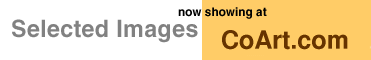[ Graphics Archive | Up | Comments ]

### Special Topics:Computational Geometry

Qhull 4-d cube by Brad BarberThis is the approximate convex hull of 10,000 points that are randomly distributed in a 4-d hypercube. Each polygon in the picture corresponds to a ridge or 4-d edge of the convex hull. The black lines are Geomview's bounding box. Geomview is displaying this 4-d object as if it were in 3-d.

A hypercube has eight facets, two for each coordinate axis. Each facet is a cube. A ridge is the intersection of two facets. Each ridge of the hypercube is a square.

A hypercube is the same as a cube, but in one dimension higher. A cube has six facets, two for each coordinate axis. Each facet is square.

The convex hull of all the points in the 4-d hypercube would be the hypercube. So if an approximate convex hull of 10,000 points in the hypercube has eight facets, it should approximate the hypercube.

An approximate convex hull has "thick" facets whose ridges are "close" to the intersection between the facets. This picture projects the vertices of the ridges to the intersection of the facets' hyperplanes. The vertices define the polygons.

This picture shows four of the eight facets in the approximate convex hull. You can see a small cube in the center. The edges do not meet up because of the approximation. The cube is the facet of the approximate convex hull that is furthest away from you. You can't see the facet that is closest because Geomview's camera is inside this facet.

You are looking at the small cube from three of the facets that are adjacent to the cube. You can see hints of the three other facets that are attached to the back side of the cube. These facets look trapezoidal because they were projected "at an angle" from 4-d. The center facet looks like a cube because it was projected "straight down" from 4-d.

The picture was generated with Qhull and Geomview. Qhull is a program for computing convex hulls, Delaunay triangulations, Voronoi vertices, and halfspace intersections.

How to make it: `rbox 10000 D4 | qhull s Gh C-0.03 C0.1 Qc >a`

Image created: April 1995The Geometry Center Home Page